﻿ DARPA2潜艇模型非定常流动粘性流场和水动力计算
 舰船科学技术2019, Vol. 41Issue (4): 19-24PDF
DARPA2潜艇模型非定常流动粘性流场和水动力计算

1. 海军航空大学青岛校区，山东 青岛 266000;
2. 海军工程大学，湖北 武汉 430033

Unsteady viscous flow and hydrodynamic force's numerical methodology of DARPA2 submarine model
YU Xiang-yang1, YAO Ling-hong1, MENG Qing-chang2, LIU Ju-bin2, ZHANG Zhi-hong2
1. Naval Aviation University Qingdao Brance, Qingdao 266000, China;
2. Naval University of Engineering, Wuhan 430033, China
Abstract: A calculating method of finite volume method which is based on unstructured meshes is proposed to simulate the unsteady flow and hydrodynamic force of DARPA2 submarine model, and the dynamic grid technique is adopted to solve the unsteady state with dynamic boundary problem. The initial variables of flow is convergent by changing the boundary conditions and part of relaxing factor. Also, the motion of submarine model is defined by UDF model, which is compiled in this paper. Initial flow is discussed with the purpose of getting the accurate unsteady results. In model-1, the imitative effect is preferable and the calculation is stable. The uncertain factor in the experiment, including equipment and the physics character of the flow variable, should be considered with a view to obtain an appropriate imitative effect．
Key words: numerical approach     unsteady viscous flow's numerical methodology     DARPA2
0 引　言

1 数值离散方法

 $\frac{{\partial {u_i}}}{{\partial {x_i}}} = 0\text{，}$ (1)
 $\begin{split} & \frac{{\partial \rho {u_i}}}{{\partial t}} + \frac{{\partial \rho {u_i}{u_j}}}{{\partial {x_j}}} = - \frac{{\partial p}}{{\partial {x_i}}} +\\ & \frac{\partial }{{\partial {x_j}}}\left[ {\mu \left( {\frac{{\partial {u_i}}}{{\partial {x_j}}} + \frac{{\partial {u_j}}}{{\partial {x_i}}}} \right) + \frac{{\partial \left( { - \rho \overline {{u'_i}{u'_j}} } \right)}}{{\partial {x_j}}}} \right]\text{。} \end{split}$ (2)

 ${G_\upsilon } = \rho {C_{b1}}{\tilde S}\tilde \nu ,\ \ {\tilde S} = \Omega + {C_\upsilon }{\rm min}(0,\ {S} - \Omega ),\ \ {\text{取}}{C_\upsilon } = 30\text{。}$ (3)

1.1 计算模型图 1 全附体DARPA2模型 Fig. 1 DARPA2 submarine model with sail and stern appendages mounted表 1 DARPA2潜艇模型主要参数 Tab.1 DARPA2 model scale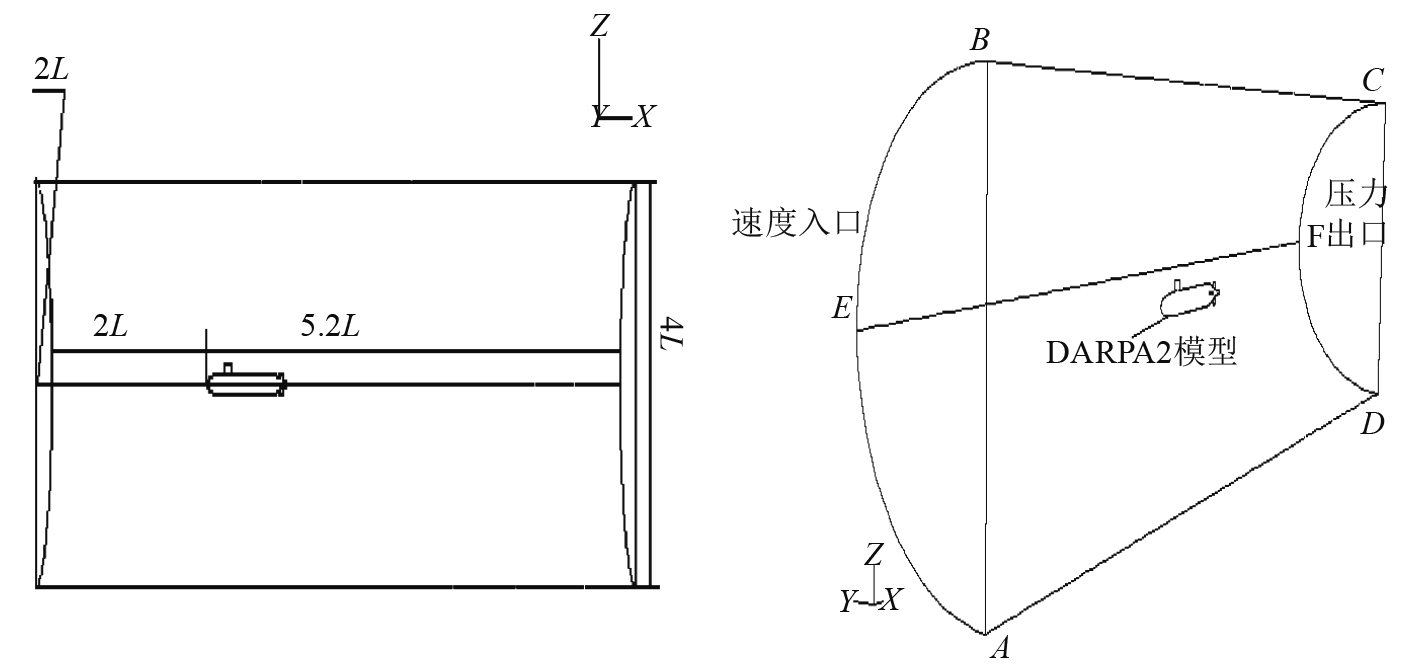图 2 模型的边界条件设置 Fig. 2 Boundary setting of the computational domain
1.2 边界条件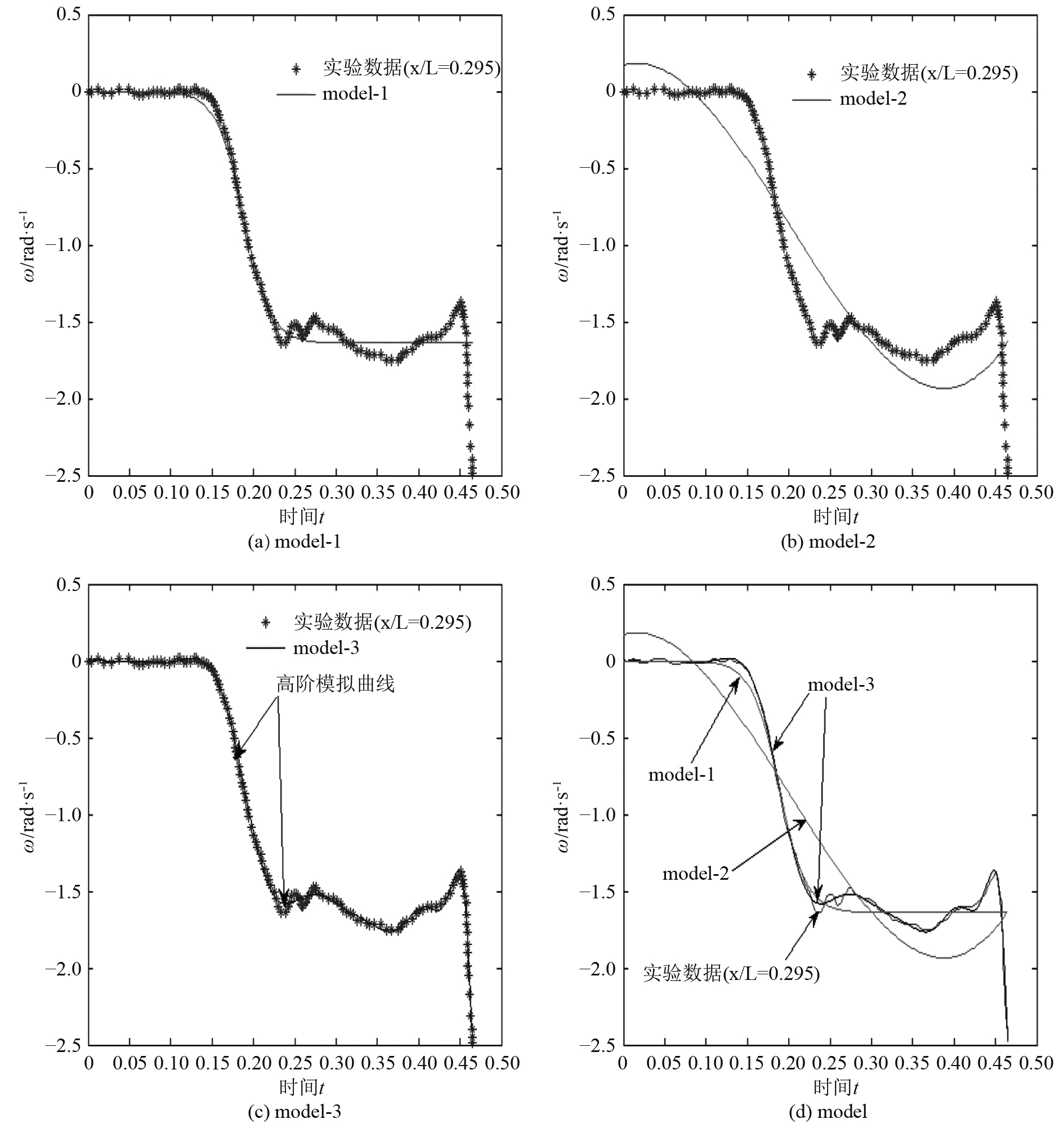图 3 角速度ω随时间变化曲线 Fig. 3 ω vs. time表 2 不同模型的角速度函数 Tab.2 The function of ω for different model
1.3 网格设置表 3 网格主要参数 Tab.3 The grids for simulation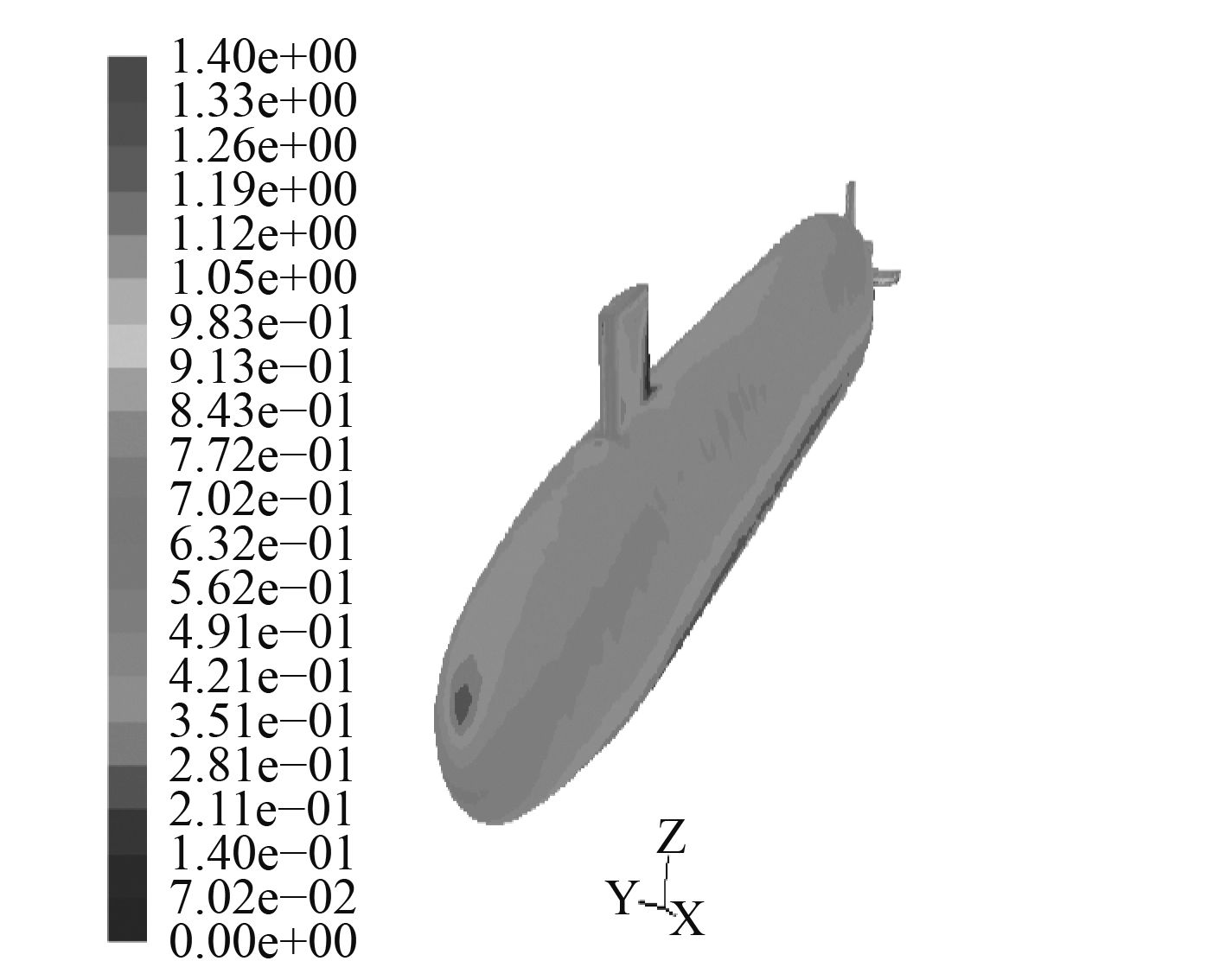图 4 攻角–25°物面 ${y^ + }$ （model-1） Fig. 4 ${y^ + }$ for the wall at –25° （model-1）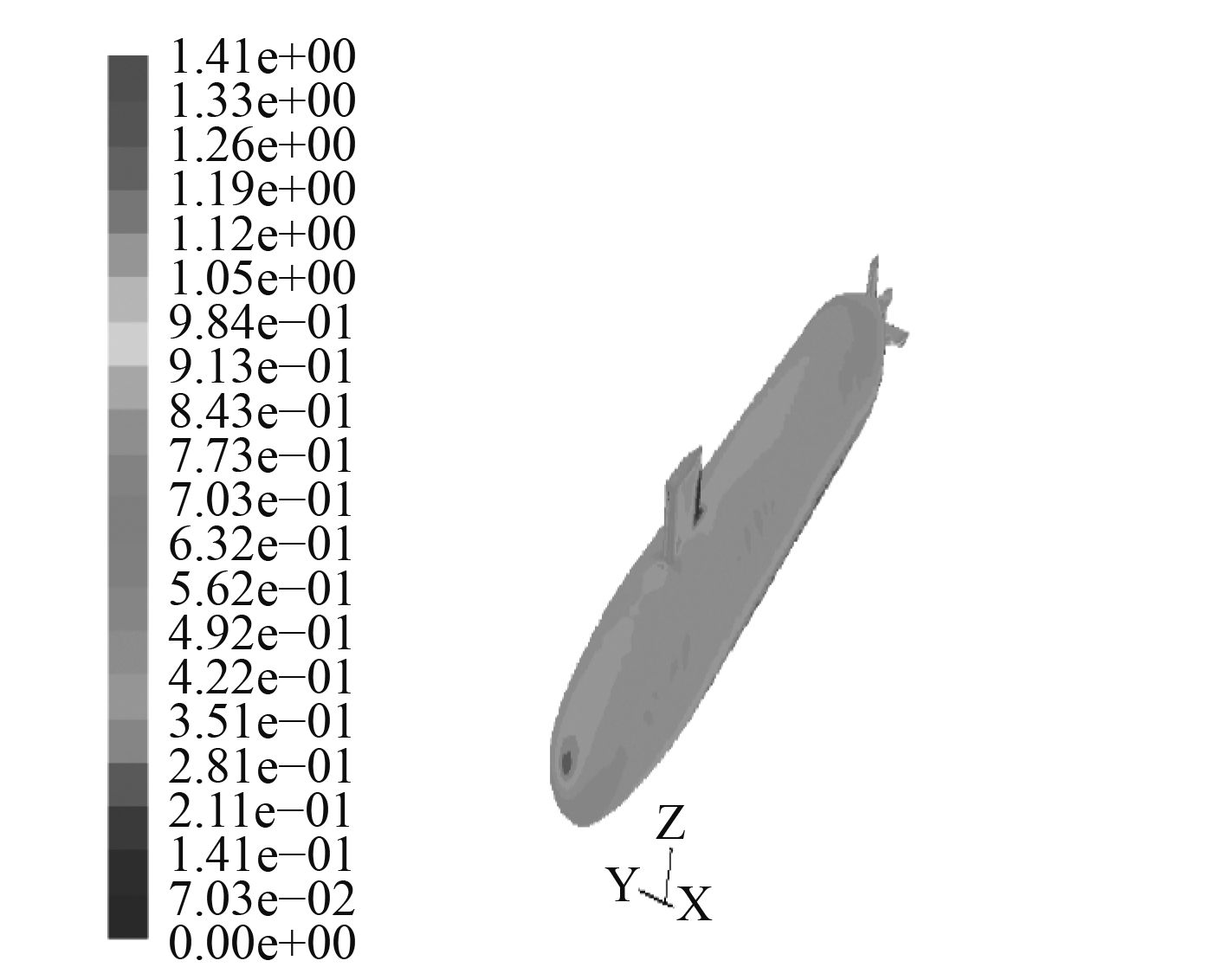图 5 攻角–25°物面 ${y^ + }$ （model-2） Fig. 5 ${y^ + }$ for the wall at –25° （model-2）

2 动态边界处理

2.1 UDF编写

2.2 网格更新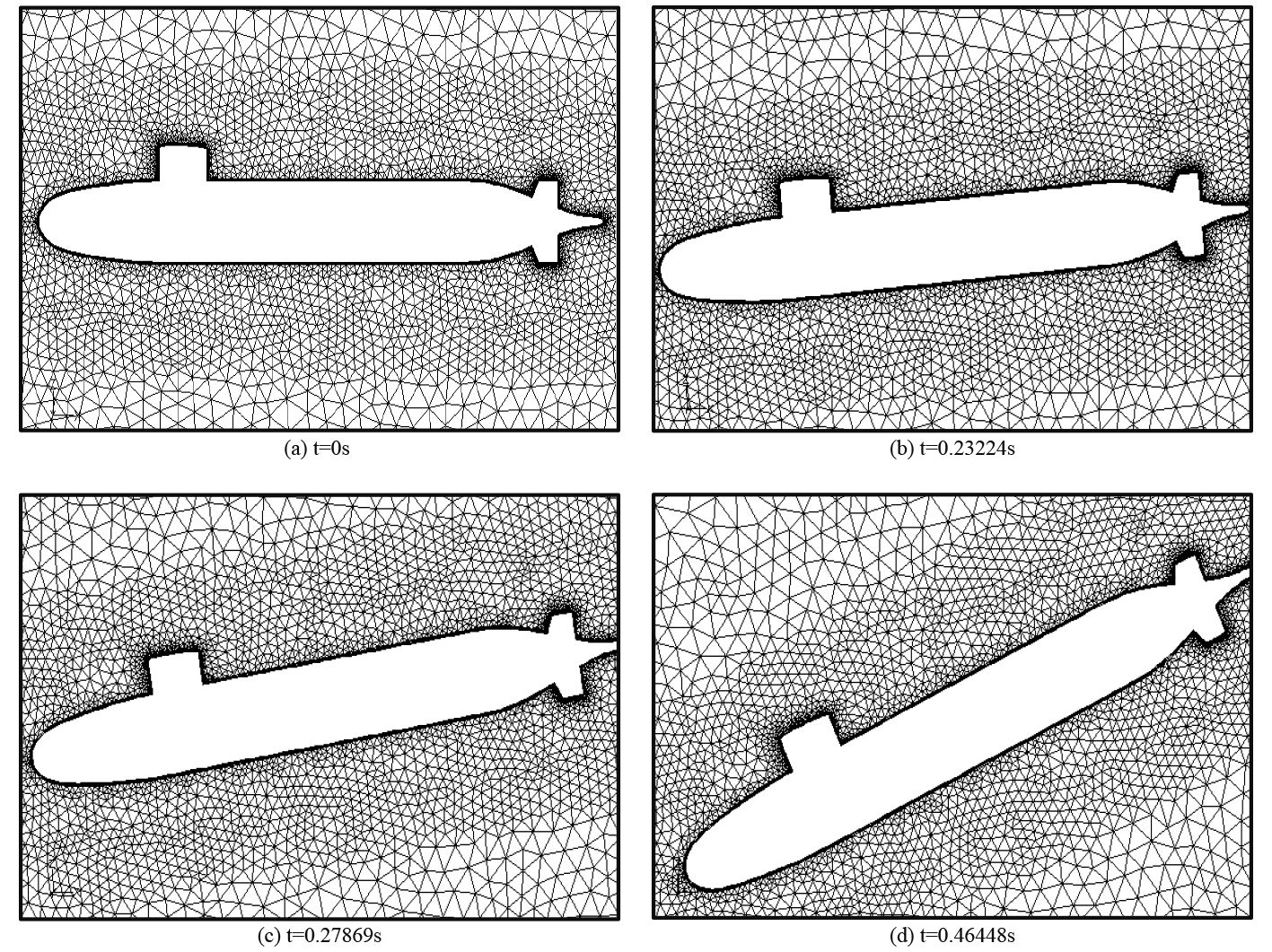图 6 网格更新示意图（model-1） Fig. 6 The view of mesh motion （model-1）

3 初始流场计算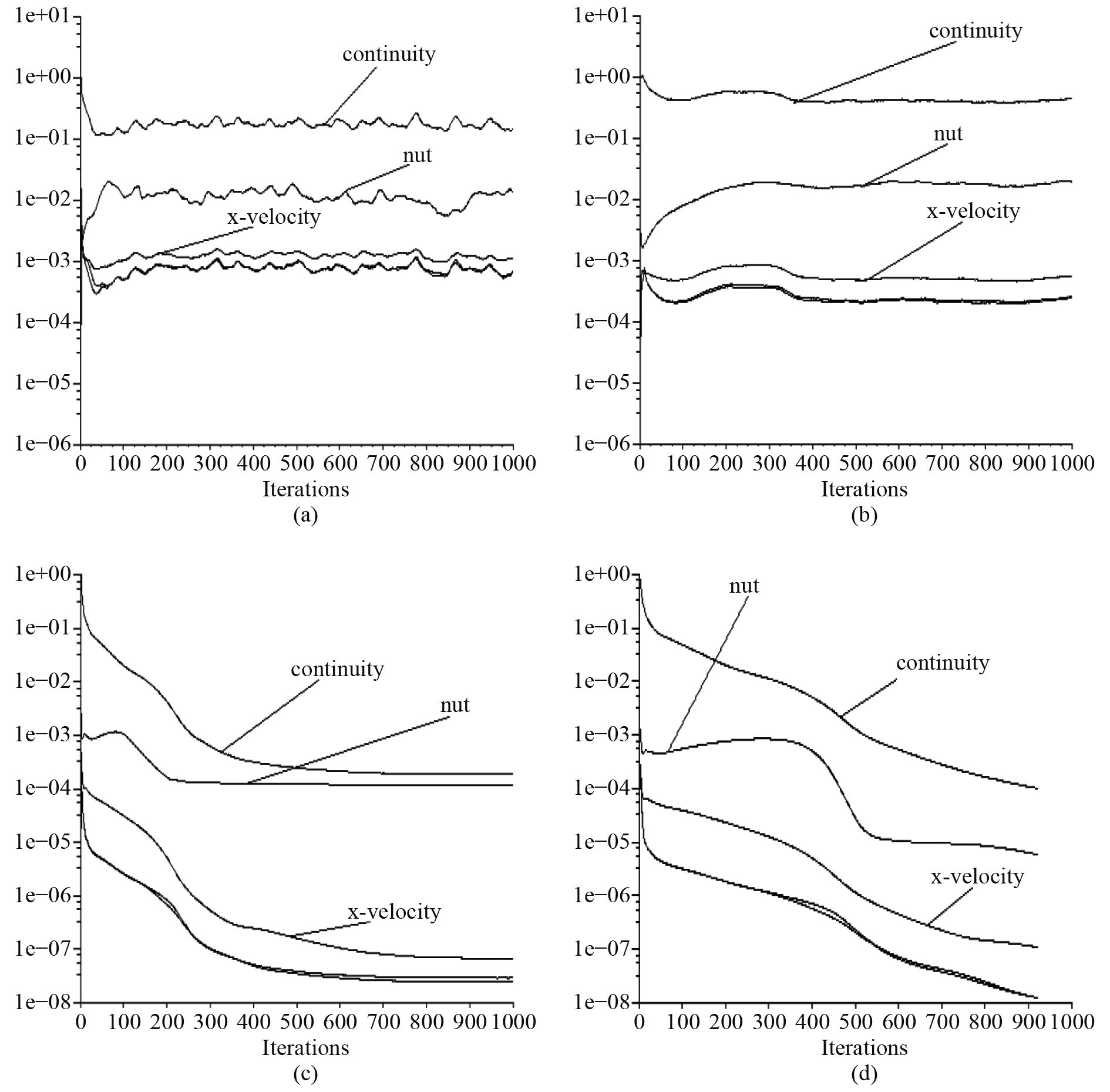图 7 初始流场变量收敛历史 Fig. 7 Convergence history of flowfied variables
4 时间步长选取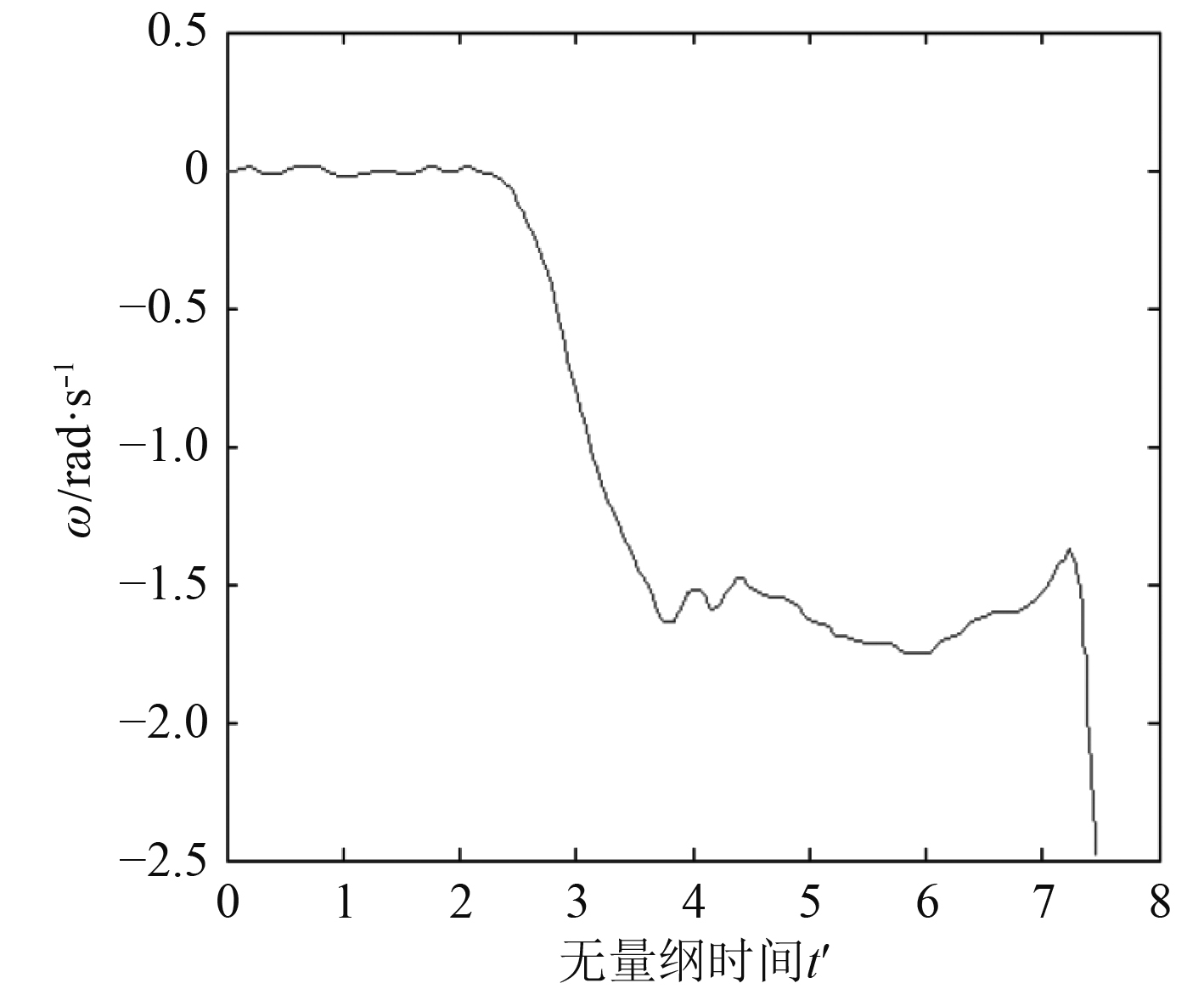图 8 角速度ω（rad/s）随无量纲时间变化曲线 Fig. 8 ω（rad/s） vs. non-dimensional time

5 计算结果分析

5.1 水动力分析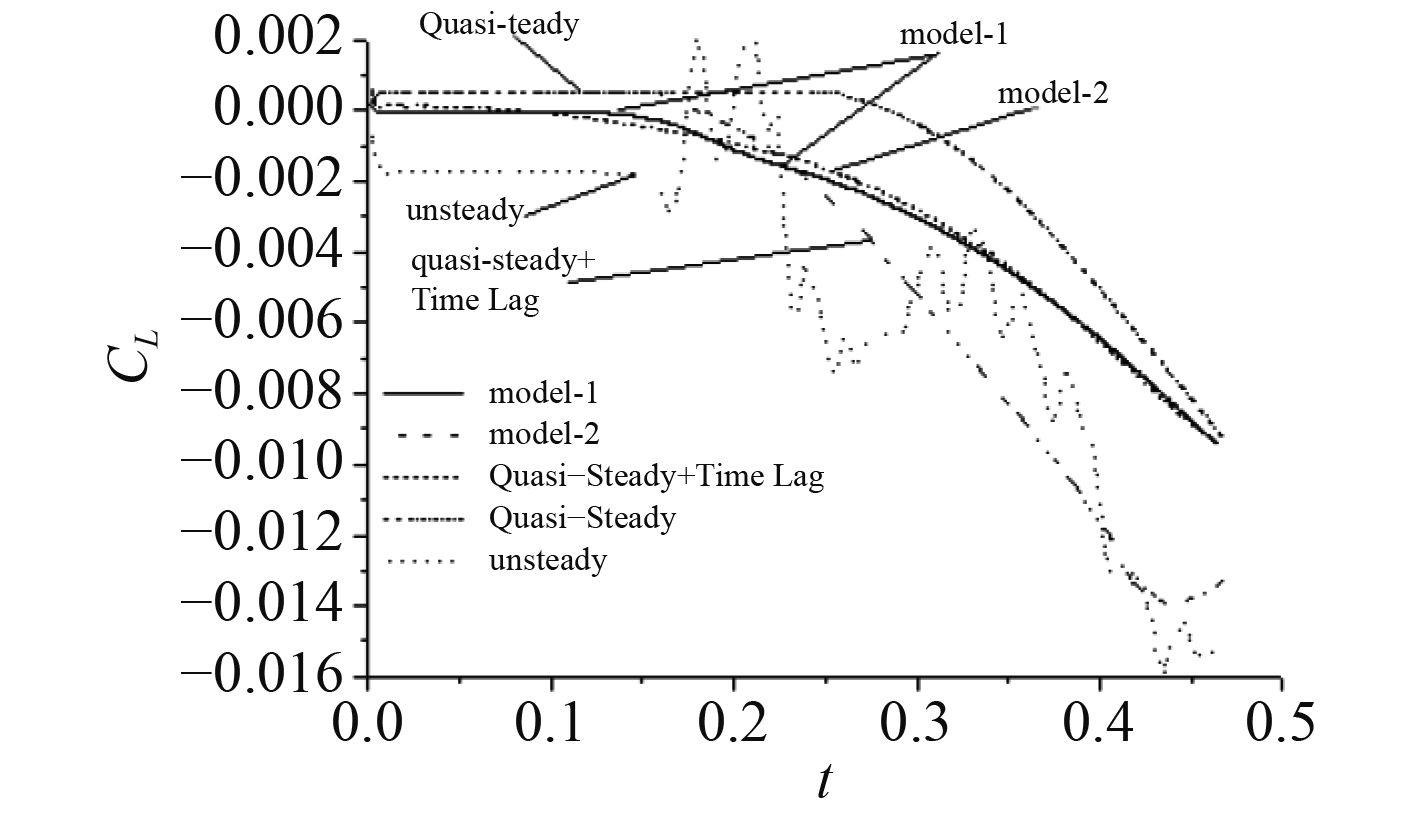图 9 升力系数与时间的关系 Fig. 9 Normal force development against time

5.2 粘性流场分析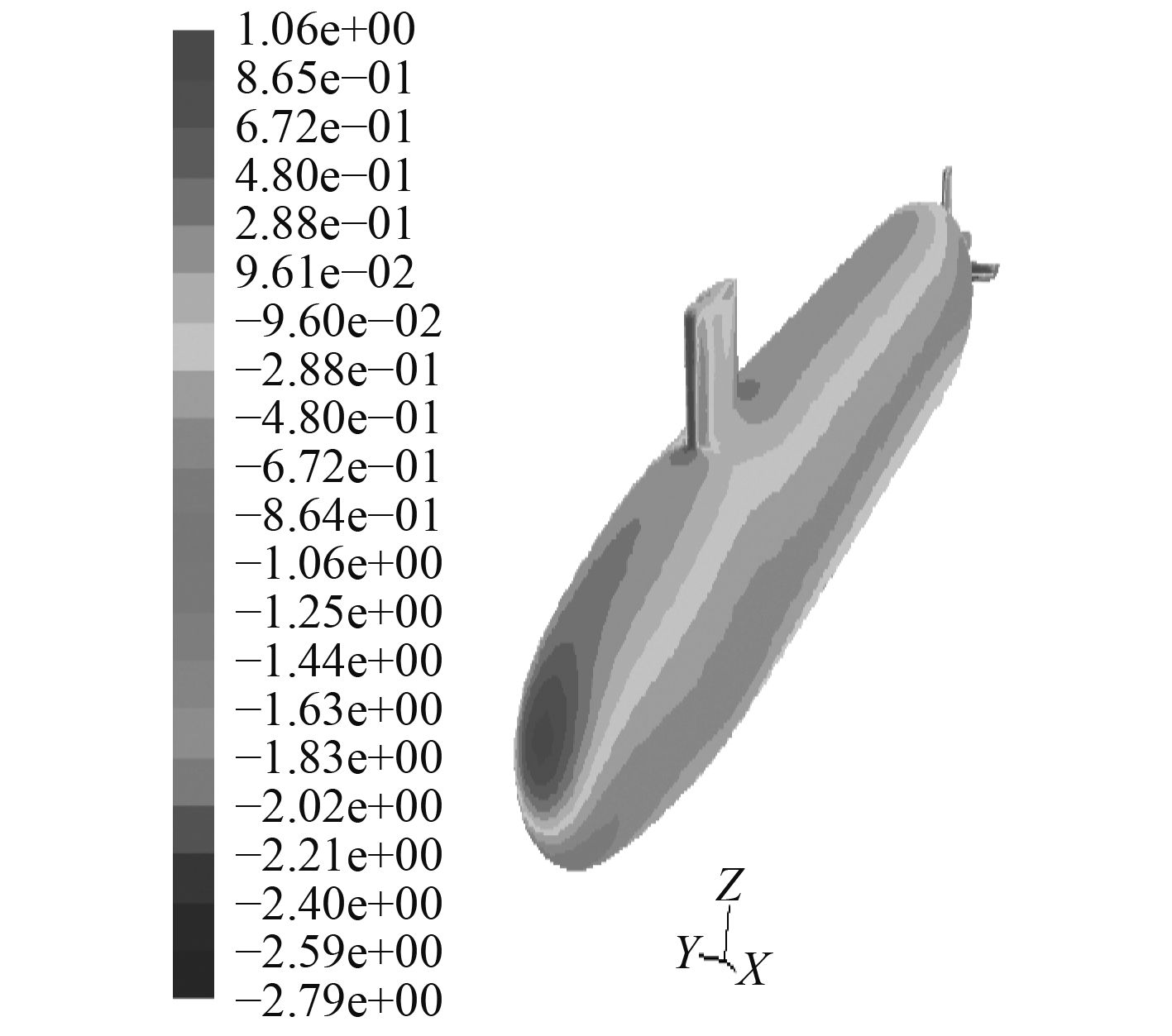图 10 攻角–25°物面压强系数（mode-l） Fig. 10 Surface pressure cofficient at –25° （mode-2）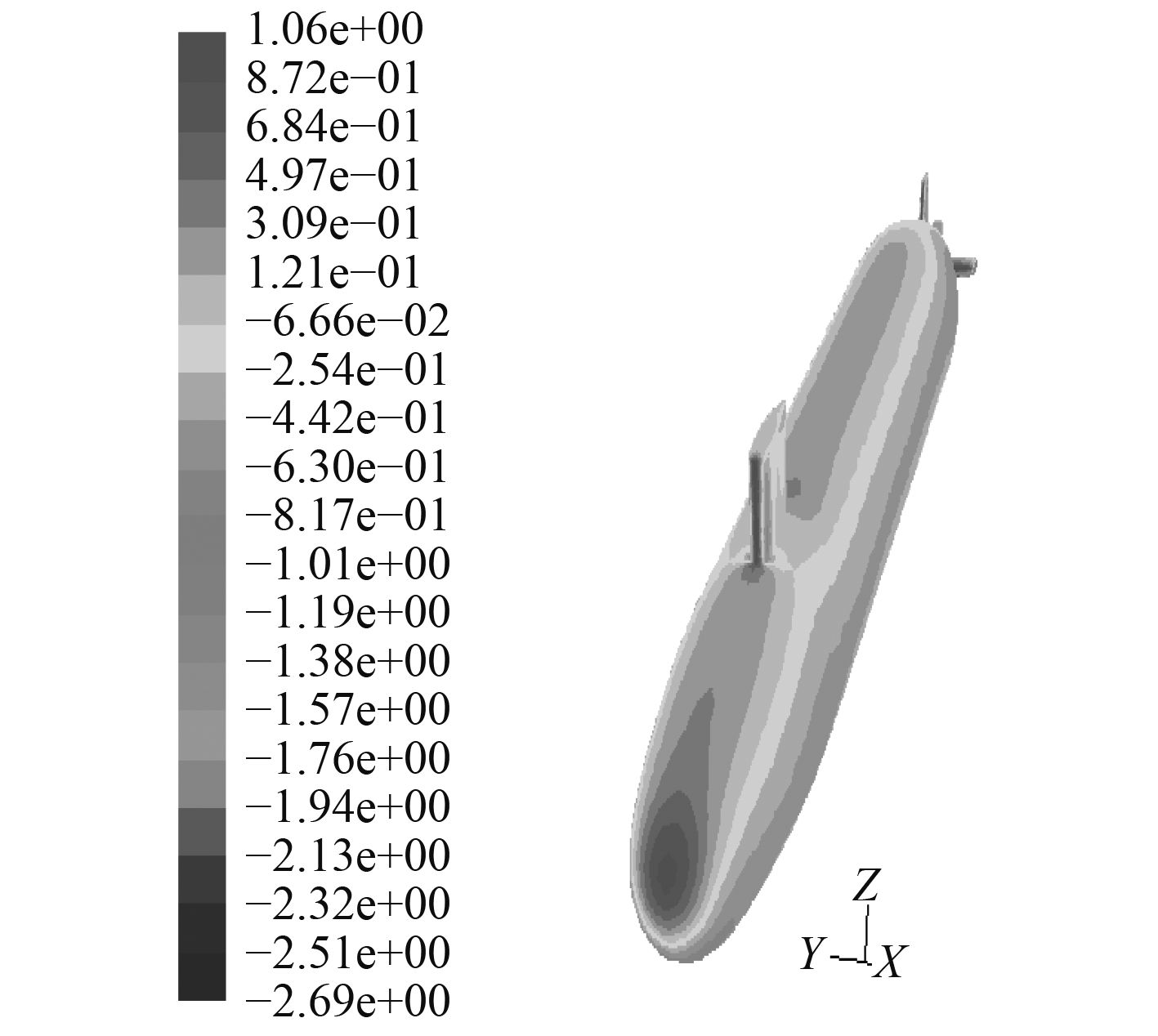图 11 攻角–25°物面压强系数（mode-2） Fig. 11 Surface pressure cofficient at –25° （mode-1）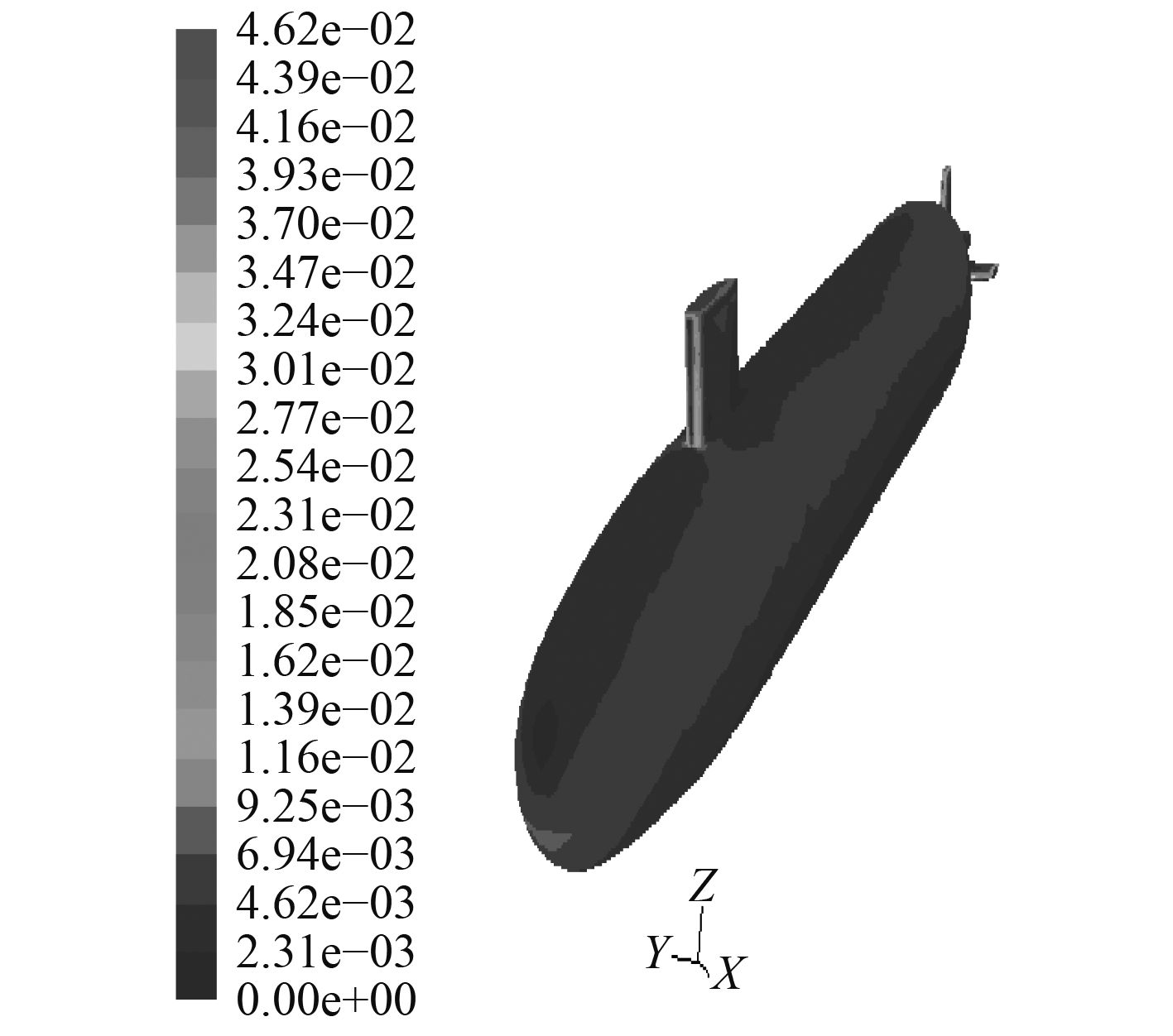图 12 攻角–25°物面剪切力系数（mode-l） Fig. 12 Surface friction cofficient at –25° （mode-l）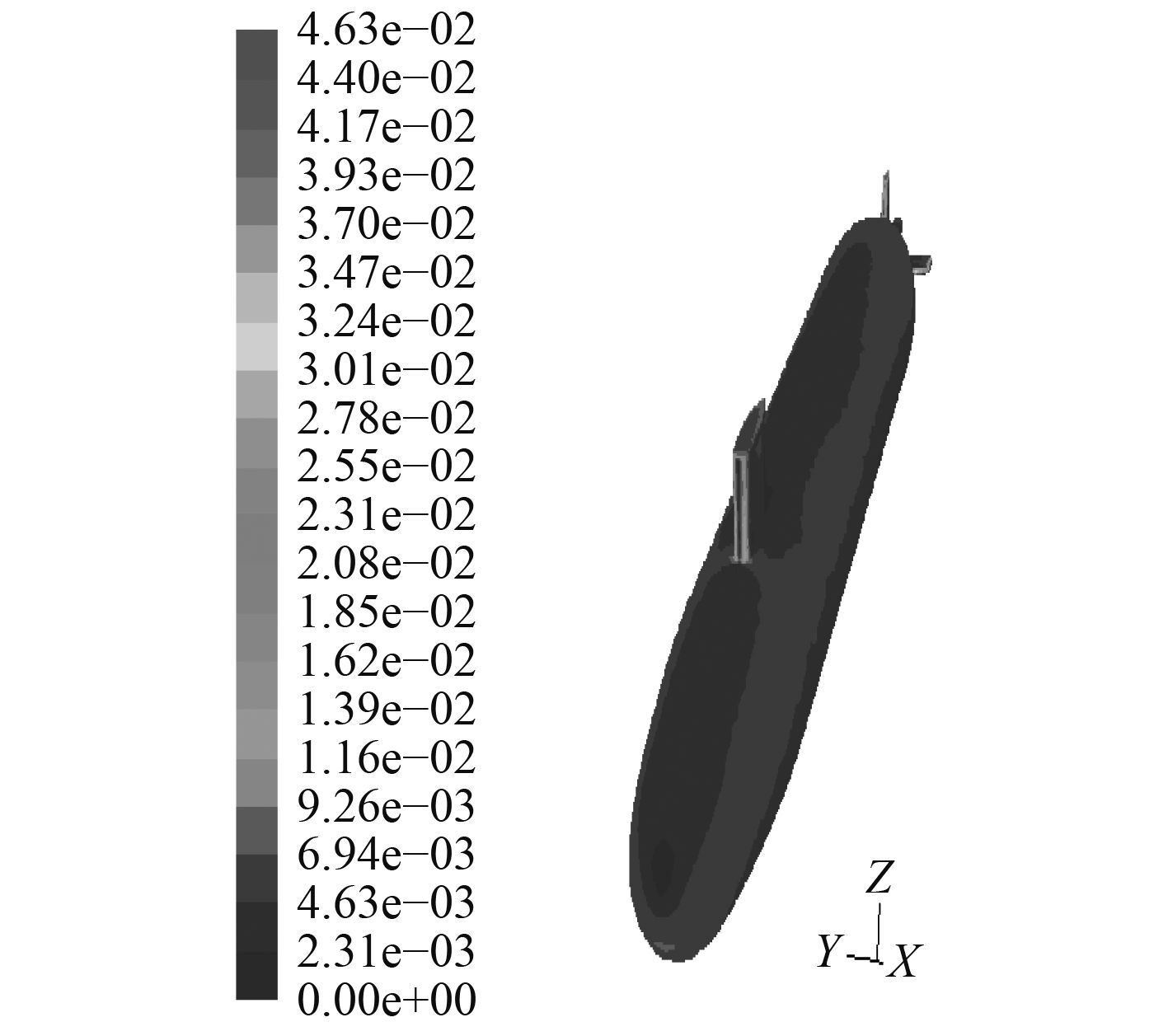图 13 攻角–25°物面剪切力系数（mode-2） Fig. 13 Surface friction cofficient at –25° （mode-2）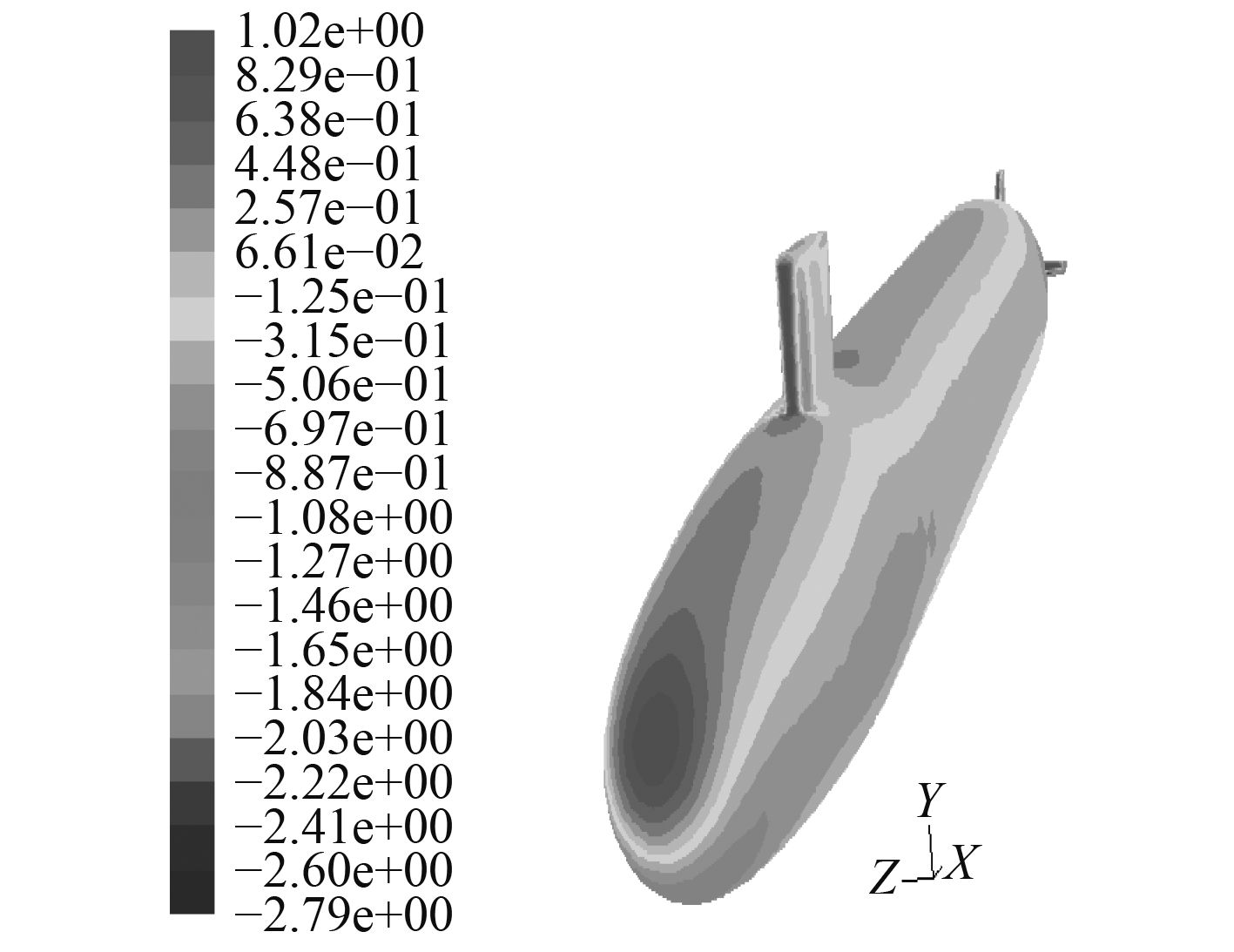图 14 攻角–25°物面压强系数 Fig. 14 Surface pressure cofficient at –25°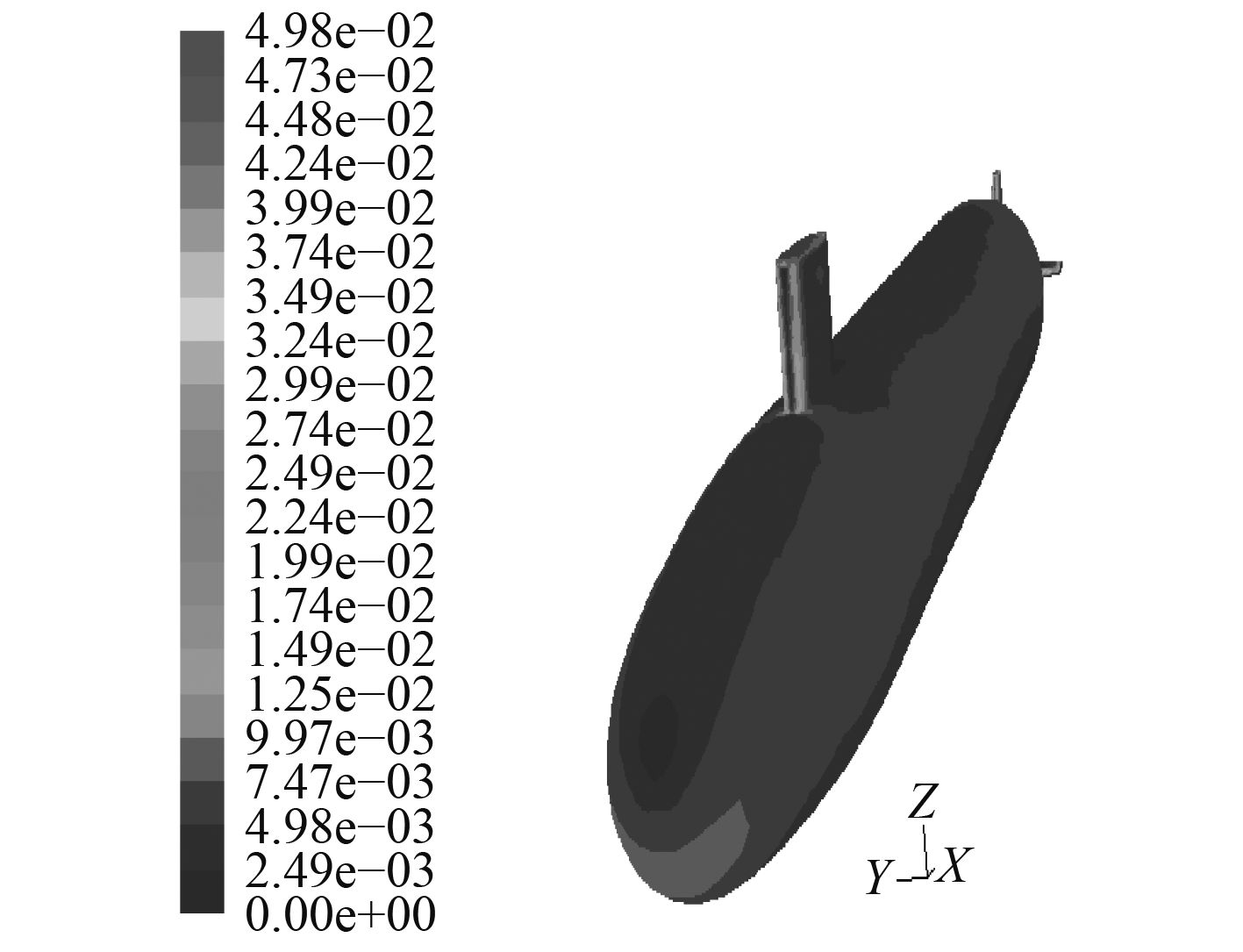图 15 攻角–25°物面切应力系数 Fig. 15 Surface friction cofficient at –25°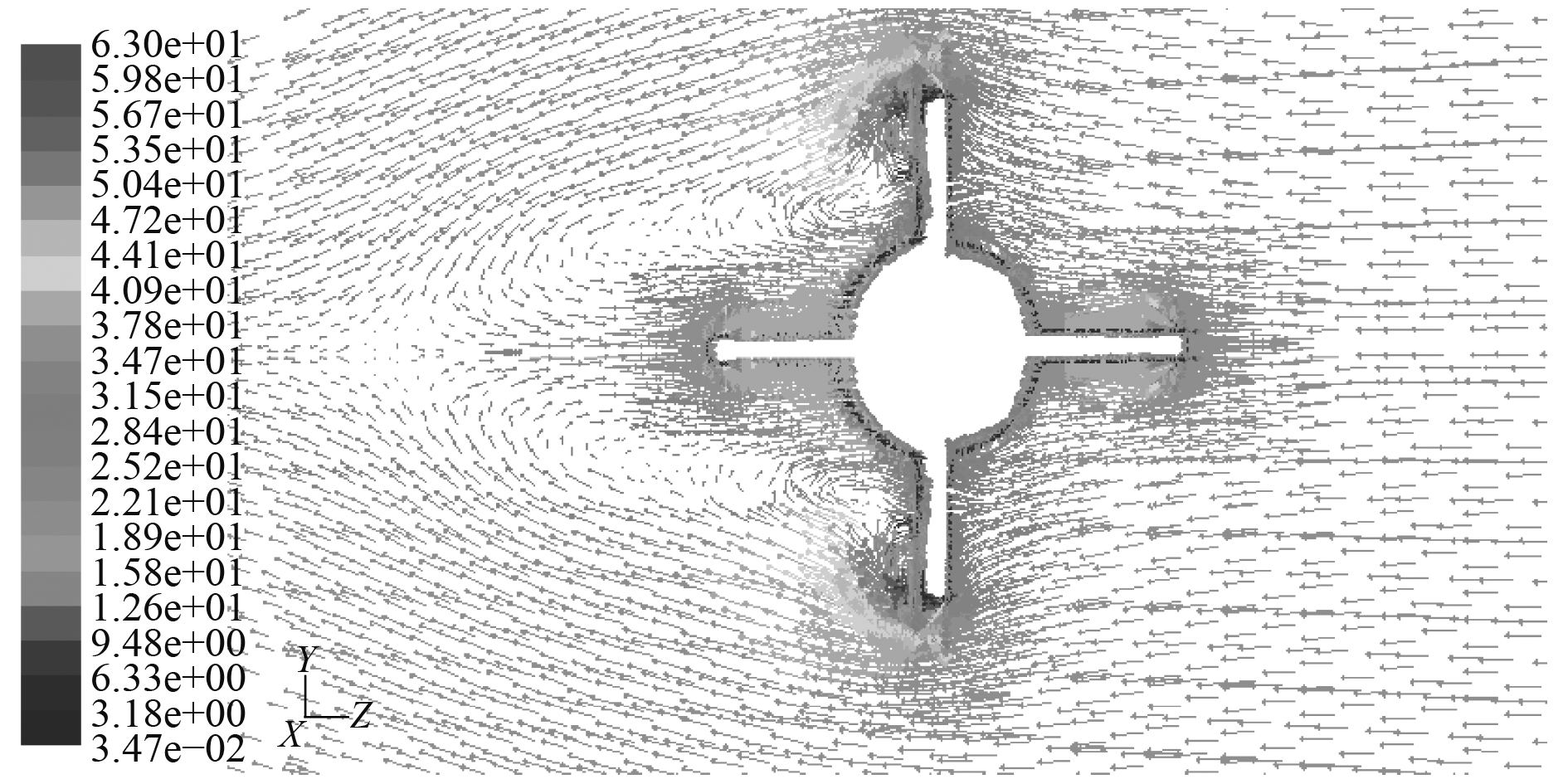图 16 攻角–25°时x/L=0.863截面速度向量图 Fig. 16 Velocity vector diagram at x/L=0.863 section and –25° angle of attack against time
6 结　语

  WHITFIELD C C. Steady and unsteady force and moment data on a DARPA2 submarine[D]. Virginia: Faculty of the Virginia Polytechnic Institute and State University, 1999.  林兆伟, 孟生, 殷洪, 等. 潜器操纵性水动力系数的数值预报方法[J]. 中国造船, 2016, 57(1): 59-68. DOI:10.3969/j.issn.1000-4882.2016.01.007  曹留帅, 朱军, 黄昆仑, 等. 全附体潜艇模型回转运动流场数值模拟[J]. 海军工程大学学报, 2015, 27(04): 17-20.  PAN Y C, ZHOU Q D, ZHANG H X. Numerical simulation of rotating arm test for prediction of submarine rotary derivatives[J]. Journal of Hydrodynamics, 2015, 27(1): 68-75. DOI:10.1016/S1001-6058(15)60457-7  周广礼, 欧勇鹏, 高霄鹏. 潜艇上浮出水运动及粘性流场直接数值模拟方法[J]. 海军工程大学学报, 2017, 29(4): 86-92.  温正, 石良辰, 等. Fluent流体计算应用教程[M]. 北京: 清华大学出版社, 2009: 26–38.  孙铭泽, 王永生, 杨琼方. 潜艇操纵性数值模拟中雷诺数的影响分析[J]. 哈尔滨工程大学学报, 2012, 33(11): 1334-1340.  周广礼, 董文才, 欧勇鹏. 潜艇应急上浮六自由度运动及黏性流场数值模拟[J]. 国防科技大学学报, 2017, 39(2): 199-206.  叶金铭, 张凯奇, 于安斌. 基于STAR-CCM+的全附体潜艇尾流场数值分析[J]. 海军工程大学学报, 2017, 29(4): 53-58.  戴天奇, 姚世卫, 魏志国. 基于动网格技术的潜艇热尾流浮升规律研究[J]. 舰船科学技术, 2015, 37(5): 86-89. DOI:10.3404/j.issn.1672-7649.2015.05.018